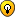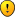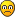## Red Wine Puzzle

Anything goes, but keep it seemly...
OK, here’s one solution for 400 ml :

A =5L cylinder
B= 3L cylinder
X the customer’s bottle

L(A), L(B) levels of A and B

3000->B
B->A
3000->B
B->A
[A=5000;B=1000]
A->0
B->A and L(A)=L(B)
[A=600;B=400]
B->0
A->B and L(A)=L(B)
[A=375;B=225]
A->X
[A=0;B=225;X=375]
B->A and L(A)=L(B)
[A=141;B=84;X=375]
B->0
B->A and L(A)=L(B)
[A=88;B=53;X=375]
A->0
B->A and L(A)=L(B)
[A=33;B=20;X=375]
B->X
[A=33;B=0;X=395]
A->B and L(A)=L(B)
[A=21;B=9;X=395]
A->0
B->A and L(A)=L(B)
[A=5;B=4;X=395]
A->X
[A=0;B=4;X=400]

JPF

PS :
1)It should be correct with a precision of 1 ml.
If the precision is not enough we can continue the process

2) same thing for 250
if A=400 and B=0
then A->B and L(A)=L(B) => [A=250;B=150]
JPF
2017 Supporter

Posts: 3755
Joined: 06 December 2005
Location: Paris, France

JPF, I think you've completely outsmarted yourself there...JPF wrote:3000->B
B->A
3000->B
B->A
[A=5000;B=1000]
A->0
B->A and L(A)=L(B)
[A=600;B=400]

At this stage could we just use the 400ml in cylinder B? So all the fuss after this and the issue about inaccuracy are totally unnecessary...But you're wrong here...If L(A)=L(B) then A=625 & B=375... Check it: 625/5000=375/3000=1/8, right?

I'll reveal all my solutions tomorrow...
udosuk

Posts: 2698
Joined: 17 July 2005

This delicious(?) (australian ?) wine makes my head spin.
It seems I’m completely drunkudosuk wrote:
JPF wrote:3000->B
B->A
3000->B
B->A
[A=5000;B=1000]
A->0
B->A and L(A)=L(B)
[A=600;B=400]

At this stage could we just use the 400ml in cylinder B? So all the fuss after this and the issue about inaccuracy are totally unnecessary...Right , udosuk.
It would be easier...

udosuk wrote:But you're wrong here... If L(A)=L(B) then A=625 & B=375... Check it: 625/5000=375/3000=1/8, right?Again right, udosuk :
1000*5/8=625
1000*3/8=375

a new attempt for 400 ml :

A =5L cylinder
B= 3L cylinder
X the customer’s bottle

L(A), L(B) levels of A and B

3000->B
B->A
3000->B
B->A
[A=5000;B=1000]
A->0
B->A and L(A)=L(B)
[A=625;B=375]
B->X
[A=625;B=0;X=375]
A->B and L(A)=L(B)
[A=391;B=234;X=375]
A->0
B->A and L(A)=L(B)
[A=146;B=88;X=375]
A->0
B->A and L(A)=L(B)
[A=55;B=33;X=375]
A->0
B->A and L(A)=L(B)
[A=21;B=12;X=375]
A->X
[A=0;B=12;X=396]
B->A and L(A)=L(B)
[A=8;B=4;X=396]
B->X
[A=8;B=0;X=400]

JPF

PS: (3/8)+(5^2*3^3/8^5)+(5*3^5/8^6)= 0,4002

I’m sure that udosuk will give a more elegant solution, but it’s all I found until now.
JPF
2017 Supporter

Posts: 3755
Joined: 06 December 2005
Location: Paris, France

Somehow I believe the cylinders being perfectly cylindrical as well as crystal clear has a bearing on this. I don't know about the level floor, but that probably is needed also.

I was thinking that somehow if a cylinder is filled, the turned at an angle to pour out enough wine to be able to see that half of it is gone you might get to 1/2 litre. (I think bisecting the cylinder at an angle where the bottom just barely is covered would do this)
Hud

Posts: 570
Joined: 29 October 2005

Hud, I think you're very close to be able to serve the picky grumpy old customer...udosuk

Posts: 2698
Joined: 17 July 2005

I suppose half of each of our containers would satisfy Mr Picky.

Somehow, I keep trying to use the displacement of the empty 3 litre cylinder by floating it on the full 5 litre cylinder, but I can't figure out what that would prove yet. It would show how much wine the empty container weighed but what to do with that info?
Hud

Posts: 570
Joined: 29 October 2005

Answer revealing time (don't try to read the tiny texts if you want to spend more time working on them yourself)...

(All measurings are done in a similar fashion: the staff first sterilize himself and the cylinders before bringing them into the isolated cellar, and then retrieve the correct amount of red wine into the 2 cylinders, and then bring them outside to pour into the containers from the customers. All excessive wine during measuring are poured back into the barrel.)

Customer 1: 4 litres

0/3 + 0/5
0/3 + 5/5
3/3 + 2/5
0/3 + 2/5
2/3 + 0/5
2/3 + 5/5
3/3 + 4/5
0/3 + 4/5
The 5L-cylinder now contains the correct amount of red wine for customer 1.

Customer 2 (the husband): 400ml

0/3 + 0/5
0/3 + 5/5
3/3 + 2/5
0/3 + 2/5
2/3 + 0/5
2/3 + 5/5
3/3 + 4/5
2.4/3 + 4/5 (pour out from 3L-cylinder and make sure both cylinders have the same liquid level)
2.4/3 + 0/5
0/3 + 2.4/5
3/3 + 2.4/5
0.4/3 + 5/5
0.4/3 + 0/5
The 3L-cylinder now contains the correct amount of red wine for customer 2.

Customer 3 (the wife): 250ml

0/3 + 0/5
3/3 + 0/5
0/3 + 3/5
3/3 + 3/5
2.25/3 + 3.75/5 (pour from 3L-cylinder to 5L-cylinder and make sure both cylinders have the same liquid level)
2.25/3 + 0/5
0/3 + 2.25/5
3/3 + 2.25/5
0.25/3 + 5/5
0.25/3 + 0/5
The 3L-cylinder now contains the correct amount of red wine for customer 3.

Customer 4 (the picky old man): 4 litres (no extra pouring)

After you fill more than half of each cylinder, then tilt it at an angle, such that some of the liquid flows back out. Carefully balance the cylinder, such that the liquid level forms an exact diagonal across the midway-rectangular cross-section of the cylinder (see this pic: http://img80.imageshack.us/img80/5315/redwinehh5.png). Now the cylinder must contain exactly half of it's capacity. Do this for both cylinders, and you get 1.5L and 2.5L of red wine respectively.
The 3L- and 5L-cylinders combined now contain the correct amount of red wine for customer 4.Hud essentially got the answer for customer 4 (Mr. Picky)...
JPF found the right concept to serve the couple, but didn't work out the correct scheme...
MCC's original method to serve customer 1 is right (and no pouring back to the barrel), but it takes 2 trips into the cellar...
udosuk

Posts: 2698
Joined: 17 July 2005

udosuk wrote:JPF found the right concept to serve the couple, but didn't work out the correct scheme...

You should say :
He didn’t work out the shorter scheme.

Even your proposed solution doesn’t guaranty exactly 400 ml for the customer.
Let’s assume that we can judge that the 2 levels are the same with an error Δh equal to 1mm (which is very accurate).
The error on the volume (i.e. 2400 ml) will be ΔV=S.Δh, S being the section of the 3L cylinder.
In order to give to the customer 400 ml with a maximum error of 1 ml, you need : ΔV=S.Δh < 1 (cm3)
with Δh=1 mm = 0.1 cm,
therefore S has to be less than 1/0.1 = 10 cm2.

For the 3L cylinder, it means a height of 3000/10 = 300 cm=3mFor the 5L cylinder, it would give 5mOn a practical matter, assuming a 30 cm height for each cylinder, we get S=3000/30=100 cm2 for the small one.
And ΔV=100 x 0.1=10 ml

You can’t guaranty better than a range 390 - 410 ml.

Now,
If we assume that we have a laser-type instrument to avoid any uncertainty on the levels of the liquids in the 2 cylinders, I think that we can conjecture that we can serve any ml (up to 3000 ml ?) to a customer.

Ex : 733
1000=625+375
X=625
375=234+141
141=88+53
X=X+88=713
53=33+20
X=X+20=733

JPF
JPF
2017 Supporter

Posts: 3755
Joined: 06 December 2005
Location: Paris, France

Bear in mind, this is a logical maths puzzle... You're taking all the fun away when you throw in the physics and calculus and such... Have you heard of the term "discrete maths"?If you want to consider error and such, even the 1st customer's solution doesn't guarantee an accurate answer... When you want to "fill up the container", in fact the liquid level could be 1mm away from the top which gives you a discrepancy of several mls...

Plus, I assumed the floor was "perfectly level and flat", and the containers were "perfectly cylindrical"... In a practical real world these things don't exist (the Earth is an almost-spherical body)...So I meant to have everything done in a "theoretical world" rather...

I don't like your solution because you're doing round-offs which gives us "theoretically definite inaccuracy" in our no-physics-only-pure-logical-mathematics-imaginary-utopia...In a practical world it could be what people do, because the margin of errors is acceptable. But as an answer to a brain teaser it's not acceptable because theoretically it couldn't give you a definitely accurate answer...

For example in the last example you gave:
JPF wrote:Ex : 733
1000=625+375
X=625
375=234+141
141=88+53
X=X+88=713
53=33+20
X=X+20=733

When you had 375=234+141, in fact it should be 375=234.375+140.625... So all the subseqent moves are all inaccurate and are no good...To get a "theoretically accurate" result, we could do this:
Code: Select all
`3/3 + 0/50/3 + 3/53/3 + 3/51/3 + 5/51/3 + 0/50.375/3 + 0.625/5 -> give 0.625 to customer0/3 + 5/53/3 + 2/50/3 + 2/52/3 + 0/52/3 + 5/53/3 + 4/50/3 + 4/51.5/3 + 2.5/53/3 + 2.5/50.5/3 + 5/50.5/3 + 0/50/3 + 0.5/50.3/3 + 0.5/50.3/3 + 0/50/3 + 0.3/50.18/3 + 0.3/50.18/3 + 0/50/3 + 0.18/50.108/3 + 0.18/5 -> give 0.108 to customer`

There's probably a shorter solution...udosuk

Posts: 2698
Joined: 17 July 2005

Previous

Return to Coffee bar